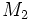# Normality is not upper join-closed for algebra loops

ANALOGY BREAKDOWN: This is the breakdown of the analogue in algebra loops of a fact encountered in group. The old fact is: normality is upper join-closed.
View other analogue breakdowns of normality is upper join-closed|View other analogue breakdowns from group to algebra loop

## Statement

It is possible to have the following: an algebra loop$L$, a subloop$N$ of$L$, two subloops$M_1, M_2$ of$L$ containing$N$, such that$N$ is a normal subloop in both$M_1$ and$M_2$ but$N$ is not a normal subloop of the subloop generated by$M_1$ and$M_2$.

In fact, we can construct a counterexample$L$ that is an Alternative loop (?), and in fact, is a Moufang loop (?).